## 2017年11月17日 星期五

### CNN using keras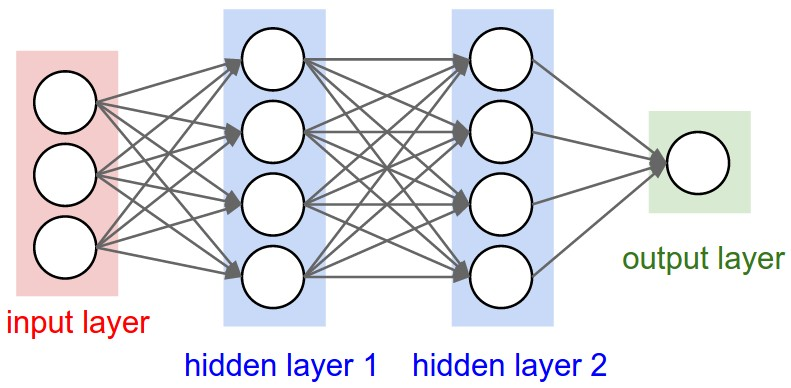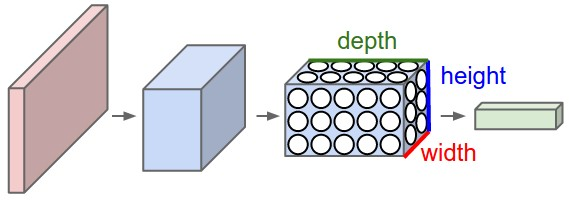A ConvNet arranges its neurons in three dimensions (width, height, depth), as visualized in one of the layers. Every layer of a ConvNet transforms the 3D input volume to a 3D output volume of neuron activations. In this example, the red input layer holds the image, so its width and height would be the dimensions of the image, and the depth would be 3 (Red, Green, Blue channels).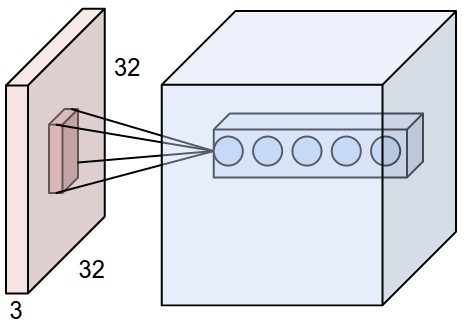An example input volume in red (e.g. a 32x32x3 CIFAR-10 image), and an example volume of neurons in the first Convolutional layer. Each neuron in the convolutional layer is connected only to a local region in the input volume spatially, but to the full depth (i.e. all color channels). Note, there are multiple neurons (5 in this example) along the depth, all looking at the same region in the input - see discussion of depth columns in text belowhe neurons from the Neural Network chapter remain unchanged: They still compute a dot product of their weights with the input followed by a non-linearity, but their connectivity is now restricted to be local spatially.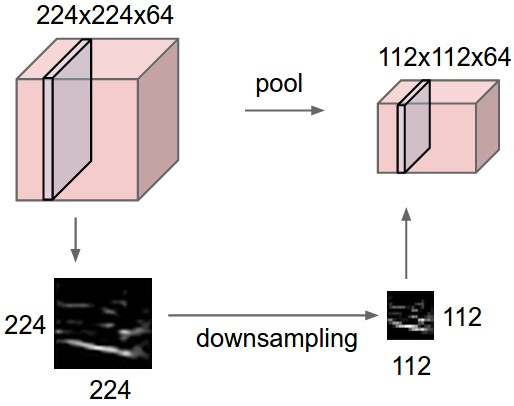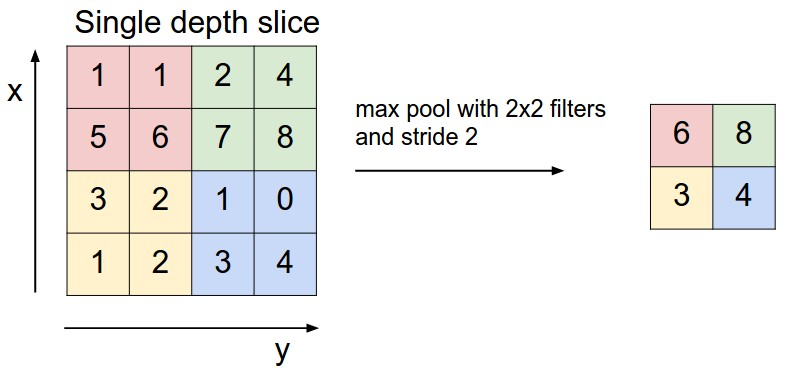Pooling layer downsamples the volume spatially, independently in each depth slice of the input volume. Left: In this example, the input volume of size [224x224x64] is pooled with filter size 2, stride 2 into output volume of size [112x112x64]. Notice that the volume depth is preserved. Right: The most common downsampling operation is max, giving rise to max pooling, here shown with a stride of 2. That is, each max is taken over 4 numbers (little 2x2 square).

# The data, shuffled and split between train and test sets:
(x_train, y_train), (x_test, y_test) = cifar10.load_data()
print('x_train shape:', x_train.shape)
print('y_train shape:', y_train.shape)
print(x_train.shape, 'train samples')
print(x_test.shape, 'test samples')

# Convert class vectors to binary class matrices.
y_train = keras.utils.to_categorical(y_train, num_classes)
y_test = keras.utils.to_categorical(y_test, num_classes)

model = Sequential()
print('input_shape:',x_train.shape[1:])

# initiate RMSprop optimizer
opt = keras.optimizers.rmsprop(lr=0.0001, decay=1e-6)

# Let's train the model using RMSprop
model.compile(loss='categorical_crossentropy',
optimizer=opt,
metrics=['accuracy'])
model.summary()

x_train = x_train.astype('float32')
x_test = x_test.astype('float32')
x_train /= 255
x_test /= 255

```_________________________________________________________________
Layer (type)                 Output Shape              Param #
=================================================================
conv2d_46 (Conv2D)           (None, 32, 32, 2)         152  ((5*5*3)+1)*2=152
其他位置也都是共用這152參數,所以不是圖愈大參數愈多
+1: bias 項, WX+b
```
```______________________________________________________________
```
```activation_67 (Activation)   (None, 32, 32, 2)         0
_________________________________________________________________
conv2d_47 (Conv2D)           (None, 30, 30, 32)        608  ((3*3*2)+1)*32=608      ```
```_________________________________________________________________
activation_68 (Activation)   (None, 30, 30, 32)        0
_________________________________________________________________
max_pooling2d_23 (MaxPooling (None, 15, 15, 32)        0
_________________________________________________________________
dropout_34 (Dropout)         (None, 15, 15, 32)        0
_________________________________________________________________
conv2d_48 (Conv2D)           (None, 15, 15, 64)        18496
_________________________________________________________________
activation_69 (Activation)   (None, 15, 15, 64)        0
_________________________________________________________________
conv2d_49 (Conv2D)           (None, 13, 13, 64)        36928
_________________________________________________________________
activation_70 (Activation)   (None, 13, 13, 64)        0
_________________________________________________________________
max_pooling2d_24 (MaxPooling (None, 6, 6, 64)          0
_________________________________________________________________
dropout_35 (Dropout)         (None, 6, 6, 64)          0
_________________________________________________________________
flatten_12 (Flatten)         (None, 2304)              0
_________________________________________________________________
dense_23 (Dense)             (None, 512)               1180160
_________________________________________________________________
activation_71 (Activation)   (None, 512)               0
_________________________________________________________________
dropout_36 (Dropout)         (None, 512)               0
_________________________________________________________________
dense_24 (Dense)             (None, 10)                5130
_________________________________________________________________
activation_72 (Activation)   (None, 10)                0
=================================================================
Total params: 1,241,474
Trainable params: 1,241,474
Non-trainable params: 0
_________________________________________________________________```